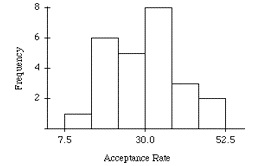### CFA Practice Question

There are 985 practice questions for this topic.

### CFA Practice Question

The following histogram represents the distribution of acceptance rates (percent accepted) among 25 business schools in 2012. In each class interval, the left endpoint is included but not the right.Which statement is true?

I. The median rate must be less than 30.
II. The first quartile must be at least 15 but no larger than 22.5.

The first quartile is the average of the 6th and 7th smallest observations. From the histogram, we know the interval 7.5 <= rate < 15 contains one observation and the interval 15 <= rate < 22.5 contains 6 observations. Thus, there are 7 observations less than 22.5, which means that both the 6th and 7th smallest observations are less than 22.5 and their average, which is the first quartile, must be less than 22.5 as well.